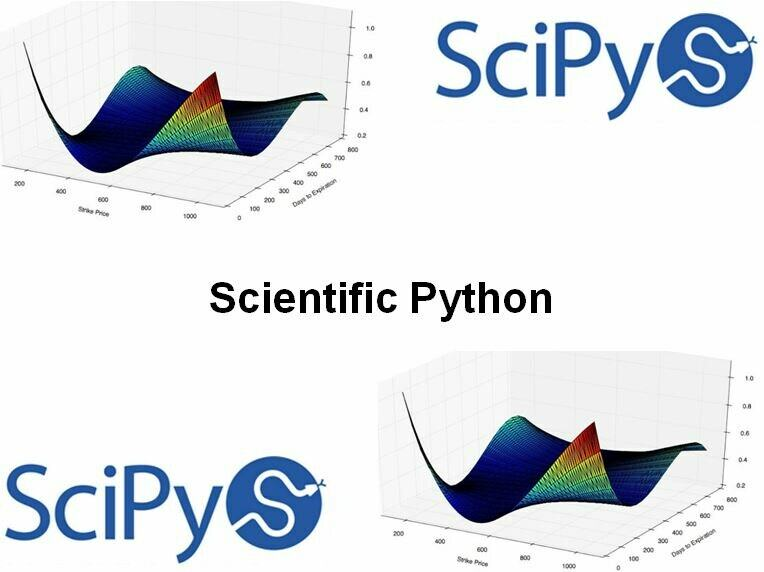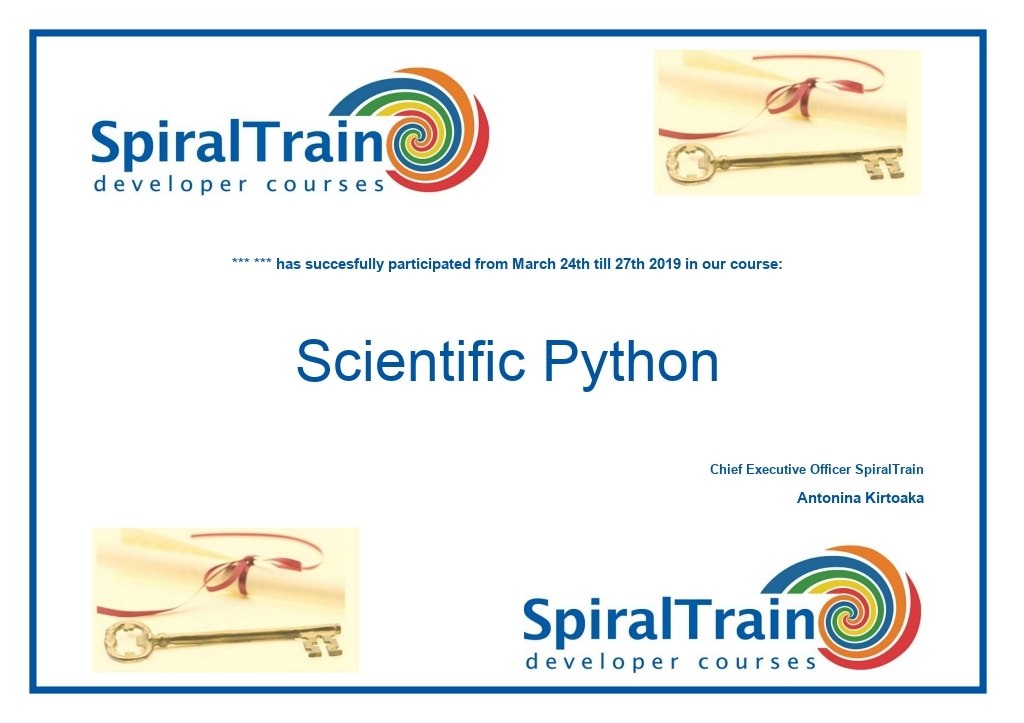# Course Scientific Python

In this course the participants will learn what can be done with the Python SciPy library for scientific computing.

Region:
2 days
€ 1299
• Content
• Training
• Modules
• General
General
• Reviews
• Certificate
• ## Course Scientific Python : Content

### Matrices in Science

The course starts with an overview of the role of matrices to solve problems in scientific computing.

### Matrix Manipulation

Next the course proceeds by reviewing basic manipulation and operations on them, followed by factorizations, solutions of matrix equations, and the computation of eigenvalues and eigenvectors.

### Interpolation and Approximation

Also interpolation and approximation is treated where advanced techniques are shown to approximate functions and their applications in scientific computing.

### Differentiation en Integration

Differentiation techniques to produce derivatives of functions are discussed as well as integration techniques showing how to compute areas and volumes effectively.

### Computational Geometry

The module Computational Geometry takes a tour of the most significant algorithms in this branch of computer science.

### Statistics and Data Mining

And finally the course pays attention to statistical inference, machine learning, and data mining.

• ## Course Scientific Python : Training

### Audience Scientific Python Course

Scientists, mathematicians, engineers and others who want to use the SciPy Python library to create applications and perform data analysis.

### Prerequisites Course Scientific Python

Knowledge of Python programming and the NumPy library is required. Some knowledge of numerical methods in scientific computing is beneficial for the understanding.

### Realization Training Scientific Python

The theory is dealt with on the basis of presentation slides. The concepts are illustrated with demos. The theory is interspersed with exercises. The course times are from 9.30 to 16.30.

### Certification Course Scientific Python

The participants get well after completion of the course, an official certificate Scientific Python.• ## Course Scientific Python : Modules

### Module 3 : Nonlinear Equations

What is SciPy
Installing SciPy stack
Anaconda distribution
Constructing matrices
Using ndarray class
Using matrix class
Sparse matrices
Linear operators
Scalar multiplication
Matrix multiplication
Traces and determinants
Transposes and inverses
Singular value decomposition
Matrix equations
Least squares
Spectral decomposition
Interpolations
Univariate interpolation
Nearest-neighbors interpolation
Other interpolations
Differentiation and Integration
Numerical differentiation
Symbolic differentiation
Symbolic integration
Numerical integration
Non-linear equations and systems
Iterative methods
Bracketing methods
Secant methods
Brent method
Simple iterative solvers
The Broyden method
Powell's hybrid solver
Large-scale solvers
Optimization
Unconstrained optimization
Constrained optimization
Stochastic methods

### Module 6 : Inference and Data Analysis

Plane geometry
Static problems
Convex hulls
Voronoi diagrams
Triangulations
Shortest paths
Geometric query problems
Point location
Nearest neighbors
Range searching
Dynamic problems
Bézier curves
Probability
Symbolic setting
Numerical setting
Data exploration
Picturing distributions
Bar plots
Pie charts
Histograms
Time plots
Scatterplots and correlation
Regression
Analysis of the time series
Statistical inference
Estimation of parameters
Bayesian approach
Likelihood approach
Interval estimation
Frequentist approach
Bayesian approach
Likelihood approach
Data mining
Machine learning
Trees and Naive Bayes
Gaussian mixture models

### Module 7 : Mathematical Imaging

Digital images
Binary
Gray-scale
Color
Alpha channels
Smoothing filters
Multivariate calculus
Statistical filters
Fourier analysis
Wavelet decompositions
Image compression
Image editing
Rescale and resize
Swirl
Image restoration
Noise reduction
• ## Course Scientific Python : General